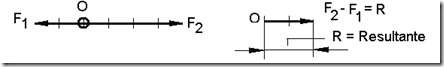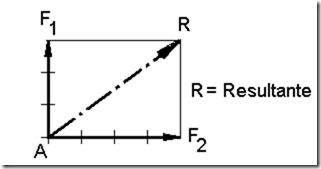Home > English > Add Style

Add the style is not the same as the sum of marbles, because they add style influenced by the magnitude and direction of force. Add two styles can be exemplified as follows.
1. The sum of two styles with the same direction and the point of capture.
Two forces each F1 = 20 N to the point capture at point A and F2 = 40 N with catching point at point B, these two forces have the same direction, namely to right horizontally, depicts both the style and specify the style number.
Scale force of 10 N # 1 cmFigure 2.15 adds style in the same direction

2. The sum of two styles with a single point of capture and in the opposite direction.
Two forces each nand F1 = 20 F2 = 40 N with the same fishing spot at the point O, these two forces have opposite direction ie F1 and F2 horizontal left to right horizontally, depicts both the style and specify the style number.
Answer: Scale force of 10 N # 1 cmFigure 2.16 adds style opposite direction

3. add two styles with one point catching and different directions.
Two forces each nand F1 = 30 F2 = 40 N with the same fishing spot at point A, these two forces have different directions, namely F1 onto F2 having a perpendicular direction to right horizontally, depicts both the style and specify the style number.
Answer: Scale force of 10 N # 1 cmFigure 2.17 adds style different directions"Tasikmalaya, Indonesia"